﻿ Parallel optimization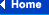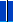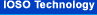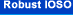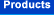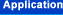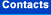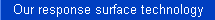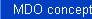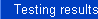### Parallel optimization

Is your model not well-parallelized? Our new algorithm of optimization process parallelization works with any model!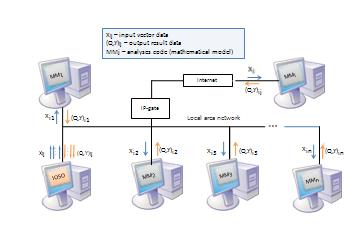The use of multiprocessor computers and clusters is one of the prospective trends improving optimization process efficiency. In this case, the reduction of elapsed (clock) computing time can be achieved by the parallelization of the computational model itself, or by adaptive organization of the optimization process for parallel computations. The first approach implies the use (or development) of mathematical models or engineering applications suitable for efficient employing of parallel processors. The latter makes it necessary to develop or to modify the corresponding optimization techniques.

We have developed the new optimization software IOSO PM, which uses parallel clusters. Our algorithm allows us to reach the speed-up parameter value that equals the total number of operational CPUs. For example, when using 20 processors we can speed-up the optimization process 40 and more times. Our algorithms allow for the most efficient usage of existing computational resources because the number of processors actively involved in solving the problem is independent of the problem dimension. For example, when solving a 10 variable problem one can employ from 1 to 100 and more processors. Our algorithms make it realistic to formulate and solve the optimization problems even when many hours are required to the response values for one combination of design variables (for example, 3D CFD codes). Combining our parallel procedures with our algorithms of multilevel optimization significantly expands the limits of solvable complex practical problems.

The main difference between the developed parallel optimization algorithm and the basic IOSO algorithm is in the information received by the data analysis and moving strategy unit. This information is not for a single point only, but for a whole set of points, the number of which is equal to the number of slave processors. This can affect the algorithm work efficiency. To evaluate this effect, a testing of the developed algorithm has been carried out.